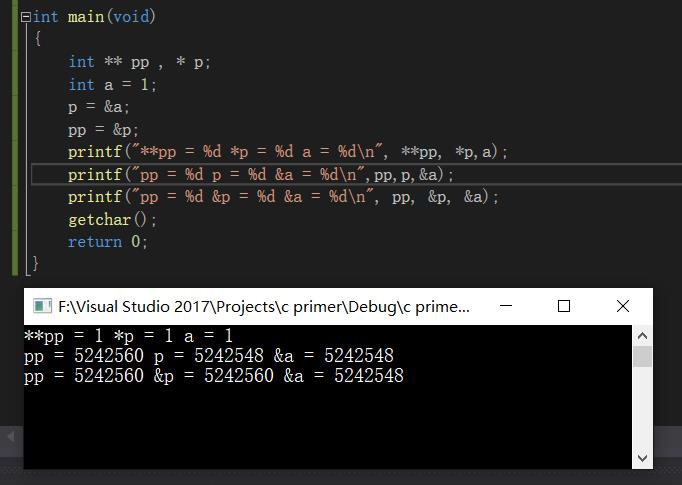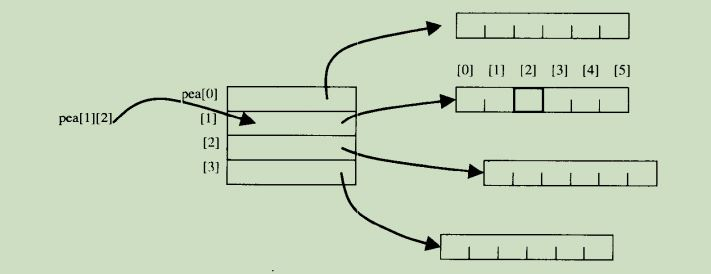## 什么时候数组和指针相同

### 规则一：表达式中的数组名就是指针

``````int a, *p, i = 2;
p = a;
p[i];
``````

### 规则2：C语言把数组下标作为偏移量

``````int a, *p, i = 2;
p = a;
for(i = 0;i < 10;i++)
{
*(p+i) = 0;  //与a[i] = 0;相同
}
``````

## 指针的指针

`&` 符号的意思是取地址，也就是返回一个对象在内存中的地址。
`*`符号的意思是取得一个指针所指向的对象。 也就是如果一个指针保存着一个内存地址，那么它就返回在那个地址的对象。

`&`：取址。
`*`：取值。## 指向数组的指针

``````char (*A);  //指向一个内含两个char类型的值的数组
char ch = {"qwert","terew","ytrytr"};
A = ch;      //使A指向ch的第一行
printf("%s\n", A);
printf("%s\n", A);
printf("%s", *(A+2));  //使用指针表示
``````

``````qwert
terew
ytrytr
``````

``````char (*p)[] = ch;
``````

## 指针数组

``````// A是一个内含n个指针元素的数组，每个元素都指向char类型的指针
char *A[n];
````````````for(j = 0;i <= 4;j++)
pea[j] = malloc(6);
``````

``````malloc(row_size*column_size*sizeof(char));
``````

### char *A[]和char **A区别

1.用`char * A []`，你不能给`A`赋值，只能赋`A[x]`;

2.用`char * A[]`，你可以初始化一个数组（例如`char * A [] = {“foo”，“bar”}`）并使用`sizeof（A）`来获取大小（64位机器，上面的例子给你16，因为它是两个`char *`的数组;用`char ** A``sizeof（A）`总是返回一个指针的大小（在64位机器上，8）。

## 可以写成`P[][]`类型的几种定义

``````int p;
int *p;
int **p;
int (*p)
``````

### 区别

1.当`char []`作为函数的参数时, 表示 `char *` 当作为函数的参数传入时, 实际上是拷贝了数组的第一个元素的地址,

`char x`; 然后调用 `test(x)` 则等同于把 `x` 的第一个元素的地址赋予给参数 `a` .

2.`char * a``char a[]`

`char *a` 则把指针存在stack，把内容存在constants.

3.`char * a``char a`

`char a ` 表示一位放10个元素, 二维放20个元素, 值存放地是一块连续的内存区域, 没有指针.

4.小窍门 : `[]``*`的数量对应, 如 `char a[][]`的指针层数是2, 相当于`char **a`; `char *a[]`也是如此, 两层指针. 迷糊的时候数数到底有几个`*`几个`[]`, 就知道什么情况下存储的是内容还是地址了? 如`char a[][]` 的情况里面: `&a`, `a`, `*a` 都是地址, `**a` 是内容.

## 参考资料

C专家编程

C和指针（第二版）微信支付宝